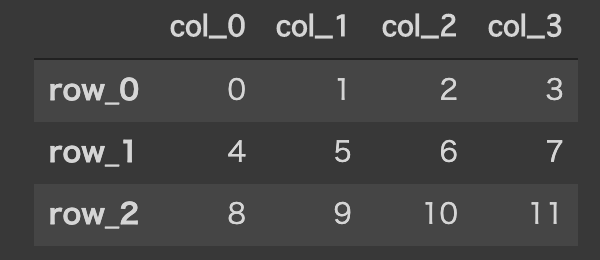Introduction

I'm using DataFrame and couldn't find a site that explains the title, so I'll share it. How to specify the default Index label.

How to use the basic at method

First, prepare a suitable DataFrame.

import pandas as pd
import numpy as np

df = pd.DataFrame(np.arange(12).reshape(3, 4),
columns=['col_0', 'col_1', 'col_2', 'col_3'],
index=['row_0', 'row_1', 'row_2'])

↓ Such a DataFrame is created.To get the value of the col_2 column of the row_1 row from this DataFrame, do as follows.

df.at["row_1", "col_2"]
# => 6

So far, the basic usage of the at method.

Main subject

But what if the Index label isn't specified?

import pandas as pd
import numpy as np

df = pd.DataFrame(np.arange(12).reshape(3, 4),
columns=['col_0', 'col_1', 'col_2', 'col_3'])

↓ This is the DataFrame.There is no Index label. (Index names are 0, 1, 2 by default)

What should I do if I want to get the values of the 1th row and col_2th column of this DataFrame? Actually, I was able to get it with the following writing style.

df.at[1, "col_2"]
# => 6

** It seems that 1 does not need""**.

df.at["1", "col_2"]
# =>error!

Please note that this will result in an error. Until now, I thought that I had to specify the index with the iat method and iloc method, but you can also specify the label with the default index!

finally

This time, I shared the method of specifying the default Index label with the at method. Thank you for reading to the end. If you have any questions, please feel free to comment.